# Problem of the day! (Analytic Geometry)

in #forhive9 months ago

A triangle is defined by the points (2,6), (-2,2) & (5,2). Determine the area of the triangle in meters if one unit is equal is equal to one meter.

Graph: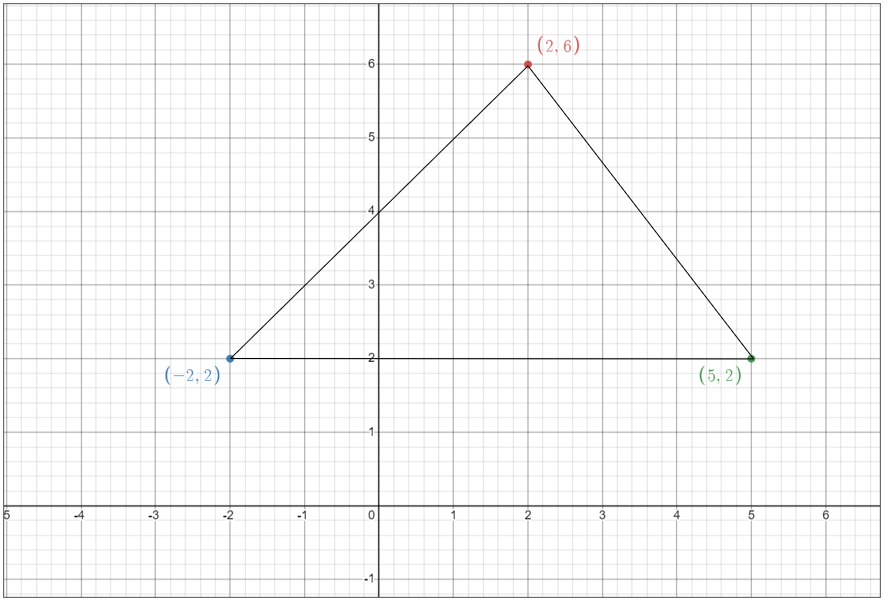Solution: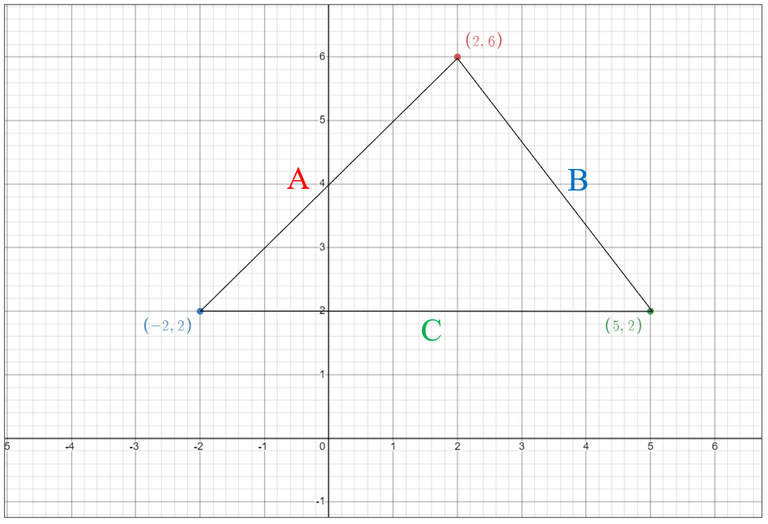Using distance formula:

A = [(-2-2)^2 + (2-6)^2 ]^0.5 = 5.657 m

B = [(2-5)^2 + (6-2)^2]^0.5 = 5 m

C = [(5-(-2))^2 + (2-2)^2]^0.5 = 7 m

Using Heron’s formula:

Area = [S(S-A)((S-B)(S-C)]^0.5

S = Semi Perimeter = (A+B+C) / 2

S = (5.657 + 5 + 7) / 2 = 8.83 m

Area = [8.83(8.83-5.657)( 8.83-5)( 8.83-7)]^0.5

Area = 14 sq. m. (Answer).

Sort:

Congratulations @exilldiogolvin! You have completed the following achievement on the Hive blockchain and have been rewarded with new badge(s):You received more than 10 upvotes.Your next target is to reach 50 upvotes.

If you no longer want to receive notifications, reply to this comment with the word `STOP`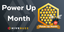The 7th edition of the Hive Power Up Month starts today!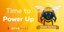Hive Power Up Day - July 1st 2022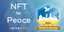NFT for peace - Thank you for your continuous support